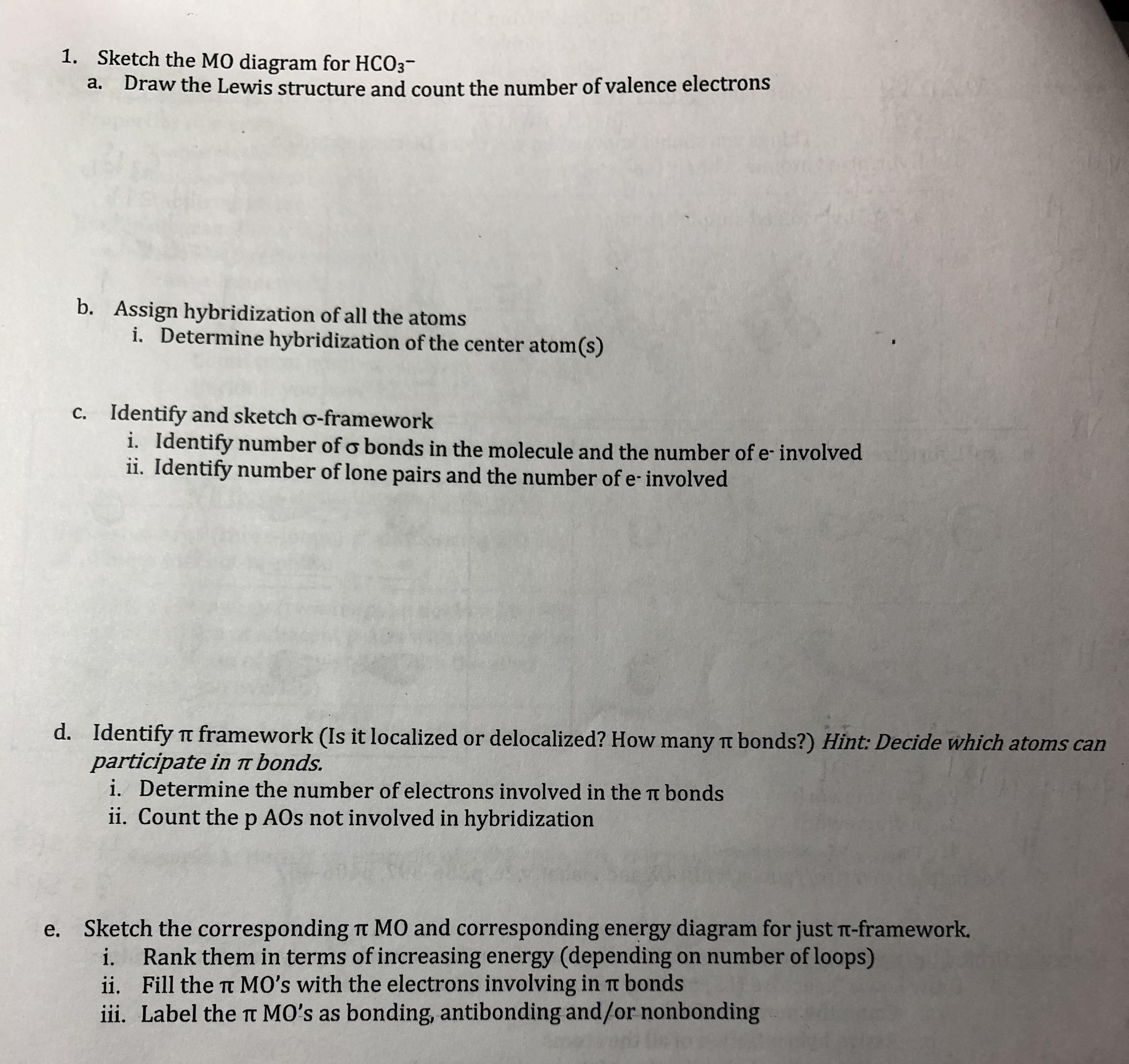# 1.Sketch the MO diagram for HCO3-a.Draw the Lewis structure and count the number of valence electronsb.Assign hybridization of all the atomsi.Determine hybridization of the center atom(s)c. Identify and sketch o-frameworki. Identify number ofo bonds in the molecule and the number of e-involvedii. Identify number of lone pairs and the number of e involvedd.Identify π framework (Is it localized or delocalized? How many π bonds?) Hint Decide which atoms canparticipate in r bonds.i. Determine the number of electrons involved in the π bondsii. Count the p AOs not involved in hybridizatione.Sketch the corresponding π MO and corresponding energy diagram for just T-framework.i. Rank them in terms of increasing energy (depending on number ofloops)ii. Fill the t MO's with the electrons involving in t bondsii. Label the t MO's as bonding, antibonding and/or nonbonding

Question
638 views

Can you help me please solve all parts of question 1? (1 a, b, c, d, and e)help_outlineImage Transcriptionclose1. Sketch the MO diagram for HCO3- a. Draw the Lewis structure and count the number of valence electrons b. Assign hybridization of all the atoms i. Determine hybridization of the center atom(s) c. Identify and sketch o-framework i. Identify number ofo bonds in the molecule and the number of e-involved ii. Identify number of lone pairs and the number of e involved d. Identify π framework (Is it localized or delocalized? How many π bonds?) Hint Decide which atoms can participate in r bonds. i. Determine the number of electrons involved in the π bonds ii. Count the p AOs not involved in hybridization e. Sketch the corresponding π MO and corresponding energy diagram for just T-framework. i. Rank them in terms of increasing energy (depending on number ofloops) ii. Fill the t MO's with the electrons involving in t bonds ii. Label the t MO's as bonding, antibonding and/or nonbonding fullscreen
check_circle

star
star
star
star
star
1 Rating
Step 1

Since we are entitled to answer upto 3 sub-parts here we are answering subparts a,b and c . Please resubmit the question to get answer of remaining subparts.

Part a.

Calculation for number of valence electrons of HCO3- ion.

Number of valence electrons of H atom = 1

Number of valence electrons of C atom = 4

Number of valence electrons of O atom = 6

Total number of valence electrons HCO3- ion = (No. of H atoms * valence electrons of H atoms) + (No. of C atoms * valence electrons of C atoms) + (No. of O atoms * valence electrons of O atoms) + number of negative charge

Total number of valence electrons HCO3- ion = 1*1+1*4+3*6+1

Total number of valence electrons HCO3- ion = 24 electrons

Lewis Structure:

Step 2

Part b.

i) Central carbon atom is bonded with two oxygen atoms by single bond, one oxygen atom by double bond and there is no lone pair on the central carbon atom so molecular geometry of HCO3- ...

### Want to see the full answer?

See Solution

#### Want to see this answer and more?

Solutions are written by subject experts who are available 24/7. Questions are typically answered within 1 hour.*

See Solution
*Response times may vary by subject and question.
Tagged in